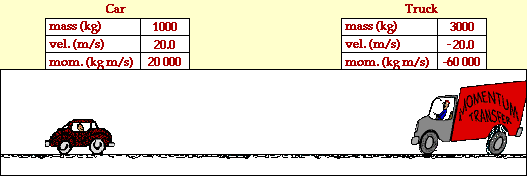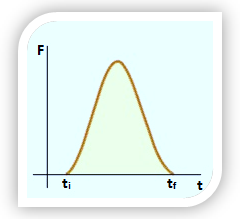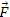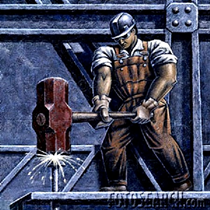Click to Chat

1800-1023-196

+91-120-4616500

CART 0

• 0

MY CART (5)

Use Coupon: CART20 and get 20% off on all online Study Material

ITEM
DETAILS
MRP
DISCOUNT
FINAL PRICE
Total Price: Rs.

There are no items in this cart.
Continue Shopping• Complete Physics Course - Class 11
• OFFERED PRICE: Rs. 2,968
• View Details

Collision

Table of Content

Types of Collision

Perfectly Elastic Collision

Inelastic Collision

Coefficient of Restitution (e)

Velocities of Colliding Bodies After Collision

Physical Quantities During a Collision

Elastic Collision

Perfectly Inelastic Collision

Elastic Collision in two Dimensions

Impulse

Conceptual Questions

Related Resources

When two bodies come close they interact with each other by one exerting force on the other. These forces are equal and opposite to each other.

A collision is said to have taken place if two bodies interact with each other and undergo a change in momentum and / or kinetic energy.

Types of Collision

(a) Perfectly Elastic Collision

A collision is said to be perfectly elastic if law of conservation of momentum and that of kinetic energy hold good during the collision.

The animation below portrays the elastic collision between a 1000-kg car and a 3000-kg truck. The before- and after-collision velocities and momentum are shown in the data tables.In the collision between the truck and the car, total system momentum is conserved. Before the collision, the momentum of the car is +20000 kg.m/s and the momentum of the truck is -60000 kg.m/s; the total system momentum is -40000 kg.m/s. After the collision, the momentum of the car is -40000 kg.m/s and the momentum of the truck is 0 kg.m/s; the total system momentum is -40000 kg.m/s. The total system momentum is conserved. The momentum change of the car (-40000 kg.m/s) is equal in magnitude and opposite in direction to the momentum change of the truck (40000 kg.m/s) .

An analysis of the kinetic energy of the two objects reveals that the total system kinetic energy before the collision is 800000 Joules (200000 J for the car plus 600000 J for the truck). After the collision, the total system kinetic energy is 800000 Joules (800000 J for the car and 0 J for the truck). The total kinetic energy before the collision is equal to the total kinetic energy after the collision. A collision such as this in which total system kinetic energy is conserved is known as an elastic collision.

Examples:-

(i) Collision between two molecules of air.

(ii) Collision of two billiard balls

(iii) Collision of two steel balls

(b) Inelastic Collision

A collision is said to be inelastic if law of conservation of momentum holds good during collision while that of kinetic energy is not.

The animation below portrays the inelastic collision between a 1000-kg car and a 3000-kg truck. The before- and after-collision velocities and momentum are shown in the data tables.In the collision between the truck and the car, total system momentum is conserved. Before the collision, the momentum of the car is +20000 kg.m/s and the momentum of the truck is -60000 kg.m/s; the total system momentum is -40000 kg.m/s. After the collision, the momentum of the car is -10000 kg.m/s and the momentum of the truck is -30 000 kg.m/s; the total system momentum is -40000 kg.m/s. The total system momentum is conserved. The momentum change of the car (-30000 kg.m/s) is equal in magnitude and opposite in direction to the momentum change of the truck (+30000 kg.m/s) .

An analysis of the kinetic energy of the two objects reveals that the total system kinetic energy before the collision is 800000 Joules (200000 J for the car plus 600000 J for the truck). After the collision, the total system kinetic energy is 200000 Joules (50000 J for the car and 150000 J for the truck). The total kinetic energy before the collision is not equal to the total kinetic energy after the collision. A large portion of the kinetic energy is converted to other forms of energy such as sound energy and thermal energy. A collision in which total system kinetic energy is not conserved is known as an inelastic collision.

Examples:-

(i) Collision between two vehicles

(ii) Collision of a rubber ball with a hard surface.

(iii) Collision of a meteorite with a planet.

A special case of inelastic collision in which the two colliding bodies stick with one other and moves as one system, is called perfectly inelastic collision.

Law of conservation of momentum holds good for perfectly inelastic collision while that of kinetic energy does not.

Examples:-

(i) Throwing a stone in wet clay.

(ii) Firing a bullet in a block of wood.

Refer this video for better understanding about types of collision:-

Coefficient of Restitution (e)

It is defined as the ratio between magnitude of impulse during period of restitution to that during period of deformation.

e = relative velocity after collision / relative velocity before collision

= v2 – v1/u1 – u2

Thus, coefficient of restitution (of  a collision), is also defined as the ratio between relative velocities of two bodies after collision to that before collision.

Case (i):-

For perfectly elastic collision, e = 1. Thus, v2 – v1 = u1 – u2. This signifies the relative velocities of two bodies before and after collision are same.

Case (ii):-

For inelastic collision, e<1. Thus, v2 – v1 < u1 – u2. This signifies, the value of e shall depend upon the extent of loss of kinetic energy during collision.

Case (iii):-

For perfectly inelastic collision, e = 0. Thus, v2 – v1 =0, or v2 = v1. This signifies the two bodies shall move together with same velocity. Therefore, there shall be no separation between them.

On the basis of coefficient of restitution, collision is divided into three types.

If e = 1, collision is called elastic if in this collision mechanical energy of the whole system is conserved.

If 0 < e < 1, collision is called inelastic.

If e = 0, collision is called perfectly inelastic.

Velocities of Colliding Bodies After Collision

In the following discussion, we will assume all the movements and velocities along a straight line. Let there be two bodies with masses m1 and m2 moving with velocities u1 and u2. They collide at an instant and acquire velocities v1 and v2 after collision. For sake of feasibility we assume that u1 > u2. Let the coefficient of restitution of the colliding bodies be 'e'. Then, applying Newton's experimental law and the law of conservation of momentum, we can find the value of velocities v1 and v2.Conserving momentum of the colliding bodies before and after the collision,

m1u1 + m2u2 = m1v1 + m2v2                                  ...... (i)

Applying Newton's experimental law, we have

v2 - v1 / u2 - u1 = -e

v2 =  v1 - e ( u2 - u1)                                             ...... (ii)

Putting (ii) in (i), we obtain

m1u1 + m2u2 = m1v1 + m2{v1 - e (u2 - u1)}

v1 = u1 (m1 - em2)/m1+m2  +  u2  (m2(1+e))/m1+m2     ...... (iii)

From (ii),

v2 = v1 - e (u2 - u1)

= u1m1(1+e)/m1+m2  +  u2  (m2-m1e)/m1+m2               ...... (iv)

When the collision is elastic; e = 1

Finally,       v1 = ( m1+m2/m1-m2 ) u1  +  ( 2m2/m1+m2 ) u2

and           v2 = ( 2m1/m1+m2 ) u1  +  ( m2+m1/m1-m2 ) u2

(i)     If m1 = m2, then

v1 = u2 and v2 = u1

When the two bodies of equal mass collide head on elastically, their velocities are mutually exchanged.

(ii)    If m1 = m2 and u2 = 0 then

v1 = -u1 and v2 = 0

(iii)    If target particle is massive (m2 >> m1) and u2 = 0, then

v1 = 0, v2 = u1

The light particle recoils with same speed while the heavy target remains practically at rest.

The u2 = 0, then v1 >> - u1 + 2u2 and v2 >> u2

(iv)   If particle is massive (m1 >> m2), then

v1 = u1 and v2 = 2u1 - u2

If the target is initially at rest (u2 = 0), then

v1 = u1 and v2 = 2u1

The motion of heavy particle is unaffected while the light target moves apart at a speed twice that of the particle.

(v)    When collision is perfectly inelastic, e = 0

v1 = v2 = [u1m1/m1+m2] +  [u2m2/m1+m2]

=  (u1m1+u2m2)/m1+m2

Physical Quantities During a Collision

When there is a collision between two bodies, and both total momentum and kinetic energy are conserved such a collision is known as an elastic collision.

Further, it is possible that some of the K.E. may be converted into some other forms of energy like heat energy. In such a case, K.E. by itself is not conserved but the total energy is conserved. Such a collision is known as an inelastic collision. Suppose the entire K.E. has changed into some other forms of energy whereas the momentum is conserved. This type of collision is called completely inelastic collision.

Elastic Collision

Here, let us analyses the simplest type of elastic collision i.e. collision of two balls moving along a straight line. This is called collision in one dimension.Consider that ball A of mass m1 moving with velocity v1 collides with another ball B of mass m2 moving with velocity v2. Conservation of momentum gives,

m1v1 + mv2 = m1v1’ + m2v2’

Conservation of K.E. gives

1/2 m1v12 + 1/2 m1v12 + 1/2 m2v2’2

If we solve both equations, we get

v1’ =  (m1-m2)/(m1+m2) v1 + 2m2/(m1+m2) v2

v'2 = 2m2/(m1+m2) v1 +  (m1-m2)/(m1+m2) v2

Bodies will move with different velocities and hence will have different kinetic energy after collision.

Perfectly Inelastic Collision

Suppose bodies A and B are undergoing a perfectly inelastic collision. In this case the two bodies stick together after collision, so that there will be a final common velocity v. Here only conservation of momentum is valid. Hence,m1v1 + m2v2 = (m1 + m2)v.

=> v = (m1v1+ m2v2)/(m1+m2) .

To analyses such collision we define a dimensionless physical quantity called coefficient of restitution as

e = |relative velocity after collision| / |relative velocity before collision| .

This is also known as Newton's experimental Law.

Note : The values of e for perfectly elastic collision is one and for perfectly inelastic collision is zero.

Elastic Collision in two Dimensions

A particle of mass m1 moving along x-axis with speed v0 makes elastic collision with another stationary particle of mass m2. After collision, the two particles move in the directions shown in the figure with speeds v1 and v2.Initial momentum of the system is

= m1 v0 î

After collision, momentum of the system is

=  (m1v1 cos q + m2v2 cos b) î + (m1v1 sin q - m2v2 sin b) j

Conserving momentum, we get

m1v0 = m1v1 cos q + m2v2 cos b                             ...... (1)

and m1v2 sin q - m2v2 sin b = 0                                      ...... (2)

From conservation of K.E.

1/2 mv02 = 1/2 m1 v12 + 1/2 m2 v22                        ...... (3)

Knowing the value of q or b, we can get the values of v1 and v2 by solving the above equations.During in an perfectly elastic collision:-

Forces involved are conservative in nature

Shape and nature of particles before and after the collision is same

During the small time of collision, deformation of two bodies does take place. The two bodies recover their original configuration completely. The energy consumed during deformation is completely restored back.

During an inelastic collision:-

Some forces involved are non-conservative in nature, like frictional force between two bodies. Work done against them cannot be restored back.

Shape of bodies is, generally, different before and after collision.

A special case of inelastic collision in which the two colliding bodies stick with one other and moves as one system, is called perfectly inelastic collision.

Law of conservation of momentum holds good for perfectly inelastic collision while that of kinetic energy does not.

Impulse

“Impulse of a force is defined as the change in momentum produced by the force and it is equal to the product of force and the time for which it acts”. Therefore, a large force acting for a short time to produce a finite change in momentum which is called impulse of this force and the force acted is called impulsive force or force of impulse.According to Newton’s second law of motion,

or,

So, Impulse of a force = change in momentum.

If the force acts for a small duration of time, the force is called impulsive force.

As force is a variable quantity, thus impulse will be,

The area under F - t curve gives the magnitude of impulse.Impulse is a vector quantity and its direction is same as the direction of.

Unit of Impulse:- The unit in S.I. system is kgm/sec or newton -second.

Dimension of Impulse:- MLT-1

Conceptual Questions

Question 1:-

Figure below shows a popular carnival device, in which the contestant tries to see how high a weighted marker can be raised by hitting a target with a sledge hammer. What physical quantity does the device measure? Is it the average force, the maximum force, the work done, the impulse, the energy transferred, the momentum transferred, or something else? Discuss your answer.The device will measure impulse. The impulse of the net force acting on a particle during a given time interval is equal to the change in momentum of the particle during that interval. Since the contestant is hitting the target with a sledge hammer the change in momentum is large and the time of collision is small, therefore it signifies that the average impulsive force will relatively large. Suppose two persons bring the harmer from the same height, but they are hitting with different forces. The person who hits with greater force for the short time interval the impulse will be more and this results the height of the mark will be more. Thus the device will measure impulse.

_____________________________________________________________

Question 2:-

Give a plausible explanation for the breaking of wooden boards or bricks by a karate punch. (See “Karate Strikes.” by Jearl D. Walker, American Journal of Physics, October 1975, p.845.)In the process, breaking of wooden boards or bricks by a karate punch, the collision between the hand and brick is only for a few milliseconds. Because the applied external force is large and the time of collision is small therefore the average impulsive force is relatively large. Thus when you break a wooden board or bricks by a karate punch you have to apply large force for the minimum time which is impulse. Therefore the impact force on the brick or wooden boards will be high.Question 1:-

A bullet in motion hits and gets embedded in a solid block resting on a frictionless table. What is conserved:

(a) momentum and kinetic energy

(b) momentum alone

(c) kinetic energy alone

(d) neither momentum nor kinetic energy?

Question 2:-

A body A strikes a body B at rest. If A and B have same masses, after the collision:

(a) A and B moves with same velocities

(b) velocity of A is greater than that of B

(c) A is at rest while B moves

(d) none of the above

Question 3:-

A body of mass m moving with velocity V collides head-on with another body of mass 2m which is initially at rest. The ratio of K.E of colliding body before and after collision will be:

(a) 8:1      (b) 7:1      (c) 6:1       (d) 9:1

Question 4:-

A ball falls from a height of 10 m on a horizontal fixed plane. After rebounding twice, it attains a height of 2.5 m. The co-efficient of restitution is:

(a) 2        (b)1           (c) 1/√2        (d) ¼

Question 5:-

Two perfectly elastic particles A and B of equal mass travelling along the line joining them with velocities 15 m/sec and 10 m/sec. After collision, their velocities will be:

A           B                                   A           B

(a) 0             25                            (b) 5          20

(c) 10           15                            (d) 20         5Q.1
Q.2
Q.3
Q.4
Q.5

b

c

d

c

c

Related Resources

You might like to refer Work, Power and Energy.

For getting an idea of the type of questions asked, refer the  Previous Year Question Papers.### Course Features

• 101 Video Lectures
• Revision Notes
• Previous Year Papers
• Mind Map
• Study Planner
• NCERT Solutions
• Discussion Forum
• Test paper with Video Solution